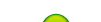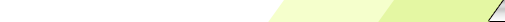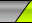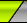Friday, 2020-02-28BioInfo PakistanHome | Sign Up | Log InWelcome Guest | RSS
Section categories
 Related Subjects This category includes brief overview of all related subjects. Defining BioInformatics In this section we tried to briefly explain what bioinformatics is ? Unviersities This contains information about universities that are offering bioinformatics degree programs. Resources Contains information about bioinformatics resources including databases, tools and techniques. Algorithms This category includes some of the basic algorithms that are usually used by bioinformaticians.
 Our poll Pakistani Student Yes No Total of answers: 0
 Chat Box Only authorized users can post messages
 StatisticsTotal online: 1 Guests: 1 Users: 0
Home » 2011 » August » 14 » Dynamic Time Warping
9:44 PM
Dynamic Time Warping

 Dynamic Time WarpingDescription:   Dynamic time warping (DTW) is an algorithm for measuring similarity between two sequences which may vary in time or speed. For instance, similarities in walking patterns would be detected, even if in one video the person was walking slowly and if in another he or she were walking more quickly, or even if there were accelerations and decelerations during the course of one observation. DTW has been applied to video, audio, and graphics — indeed, any data which can be turned into a linear representation can be analyzed with DTW. A well known application has been automatic speech recognition, to cope with different speaking speeds.   In general, DTW is a method that allows a computer to find an optimal match between two given sequences (e.g. time series) with certain restrictions. The sequences are "warped" non-linearly in the time dimension to determine a measure of their similarity independent of certain non-linear variations in the time dimension. This sequence alignment method is often used in the context of hidden Markov models.   One example of the restrictions imposed on the matching of the sequences is on the monotonicity of the mapping in the time dimension. Continuity is less important in DTW than in other pattern matching algorithms; DTW is an algorithm particularly suited to matching sequences with missing information, provided there are long enough segments for matching to occur.   The extension of the problem for two-dimensional "series" like images (planar warping) is NP-complete, while the problem for one-dimensional signals like time series can be solved in polynomial time.Example Of One Of The Many Forms Of The Algorithm   This example illustrates the implementation of dynamic time warping when the two sequences are strings of discrete symbols. d(x, y) is a distance between symbols, e.g. d(x, y) = | x - y |.```int DTWDistance(char s[1..n], char t[1..m]) { declare int DTW[0..n, 0..m] declare int i, j, cost for i := 1 to m DTW[0, i] := infinity for i := 1 to n DTW[i, 0] := infinity DTW[0, 0] := 0 for i := 1 to n for j := 1 to m cost:= d(s[i], t[j]) DTW[i, j] := cost + minimum(DTW[i-1, j ], // insertion DTW[i , j-1], // deletion DTW[i-1, j-1]) // match return DTW[n, m] }```      We sometimes want to add a locality constraint. That is, we require that if s[i] is matched with t[j], then | i - j | is no larger than w, a window parameter.   We can easily modify the above algorithm to add a locality constraint (differences marked in bold italic):```int DTWDistance(char s[1..n], char t[1..m], int w) { declare int DTW[0..n, 0..m] declare int i, j, cost for i := 0 to n for j:= 0 to m DTW[i, j] := infinity DTW[0, 0] := 0 for i := 1 to n for j := max(1, i-w) to min(m, i+w) cost := d(s[i], t[j]) DTW[i, j] := cost + minimum(DTW[i-1, j ], // insertion DTW[i , j-1], // deletion DTW[i-1, j-1]) // match return DTW[n, m] }```
Category: Algorithms | Views: 941 | Added by: Ansari | Rating: 0.0/0